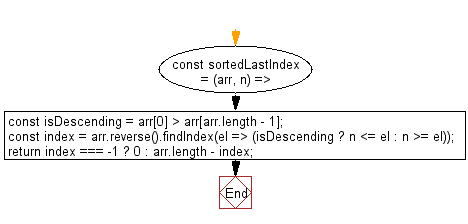# JavaScript: Get the highest index at which value should be inserted into array in order to maintain its sort order

## JavaScript fundamental (ES6 Syntax): Exercise-141 with Solution

Write a JavaScript program to get the highest index at which value should be inserted into array in order to maintain its sort order.

• Loosely check if the array is sorted in descending order.
• Use Array.prototype.reverse() and Array.prototype.findIndex() to find the appropriate last index where the element should be inserted.

Sample Solution:

JavaScript Code:

``````//#Source https://bit.ly/2neWfJ2
const sortedLastIndex = (arr, n) => {
const isDescending = arr > arr[arr.length - 1];
const index = arr.reverse().findIndex(el => (isDescending ? n <= el : n >= el));
return index === -1 ? 0 : arr.length - index;
};

console.log(sortedLastIndex([10, 20, 30, 30, 40], 30));
```
```

Sample Output:

```4
```

Flowchart:Live Demo:

See the Pen javascript-basic-exercise-141-1 by w3resource (@w3resource) on CodePen.

Improve this sample solution and post your code through Disqus

What is the difficulty level of this exercise?

Test your Programming skills with w3resource's quiz.

﻿

## JavaScript: Tips of the Day

Chunks an array into n smaller arrays

Example:

```const tips_chunkIntoN = (arr, n) => {
const size = Math.ceil(arr.length / n);
return Array.from({ length: n }, (v, i) =>
arr.slice(i * size, i * size + size)
);
}
console.log(tips_chunkIntoN([1, 2, 3, 4, 5, 6, 7,8], 4));
```

Output:

```[[1,2],[3,4],[5,6],[7,8]]
```